# Circular Linked List Implementation in C++ (with code)

Breaking into the programming world might be slightly more difficult than expected, especially when it comes to data structures and algorithms. One of the most popular data structures used throughout the world of computer science is the linked list. In this blog, we will learn about how to Circular Linked List in C++, with code for operations insertion and deletion. So, let's get started!

## What is a Circular Linked List?

A linked list has many problems on its own. One needs to store a pointer to the head of the linked list carefully somewhere and store its value inside another pointer before manipulating the linked list. To resolve this issue, we use two slightly modified versions of the linked list: the doubly linked list and the circular linked list.

A circular linked list is a linked list where all nodes are connected to form a circle. Generally, the last node of the linked list has a NULL in the address field, but a circular linked list has the address of the head node in the address field of the last node.

If you want specific code for a particular problem you got on your assignment, you can get C++ homework help from our programming experts.

If you are not up-to-date with what a linked list is; it is a data structure that allows the allocation of memory dynamically (that is, depending on our needs). It is made up of nodes that have two main components:

1. Some data inside it. It can be a combination of many data types or a single data type.
2. A pointer to the next node in the linked list.

What is the logic of a circular linked list? It is very similar to a singly linked list but the main difference is that the last node is connected to the first node. To create the list, a head pointer is set to NULL. When a new node is added, it is created with its data value. The next pointer of the new node now points to the head of the list, and the head pointer points to the new node.

Now that we know the basics, we can move forward to implementing Circular Linked List.

## C++ Implementation for Circular Linked List

The circular linked list consists of several operations that can be performed on it. We are including an implementation of the circular linked list in C++ with the various functionalities such as insertion and deletion that should come with it.

Following is the complete code of the circular linked list in C++:

```#include<bits/stdc++.h>
using namespace std;

struct Node
{
int data;								//data of the node
struct Node *next;							//pointer to the next node in the list
};

int Length(struct Node *head)						//function to calculate the length of the linked list
{
struct Node *t;
int i = 0;
if (head == NULL)								//handle underflow condition
{
return 0;
}

do
{												//handle traversal through the list
t = t -> next;
i++;
return i;
}

struct Node *Start(struct Node *head, int data)				//function to insert nodes at the beginning of the list
{
struct Node *temp = (struct Node *) malloc(sizeof(struct Node));
if (head == NULL)										//handle underflow condition
{
temp -> data = data;
}
else
{
temp -> data = data;
temp -> next = head -> next;
}
}

struct Node *End(struct Node *head, int data)						//function to insert nodes at the end of the list
{
struct Node *temp = (struct Node *) malloc(sizeof(struct Node)), *a = head;
if (head == NULL)										//handle underflow condition
{
temp -> data = data;
}
else
{
do
{
a = a -> next;
} while (a -> next != head);							//traverse to the end of the list
temp -> data = data;
temp -> next = a -> next;
a -> next = temp;
}
}

struct Node *Middle(struct Node *head, int data, int index)				//function to insert nodes anywhere in between the beginning and the end
{
if (head == NULL)								//handle underflow condition
{
cout << "List is empty!" << endl;
return NULL;
}

int len = Length(head);						//get the length of the list for making a decision
if (index > len || index < 0)						//wrong index given
{
cout << "Wrong input, insertion not possible!" << endl;
}
if (index == 0)								//insert at the beginning
{
}
if (index == len)								//insert at the end
{
}
struct Node *temp = (struct Node *)malloc(sizeof(struct Node)), *a = head;
do
{													//handle data traversal from beginning to end
a = a -> next;
} while (a -> next != head);
len = 0;
while (1)
{
if (len == index)							//handle node addition
{
temp -> data = data;
temp -> next = a -> next;
a -> next = temp;
}
a = a -> next;
len++;
}
}

struct Node *First(struct Node* head)									//function to delete node from the start of the list
{

if (head == NULL) {								//handle underflow condition
cout << "List is empty" << endl;
return NULL;
}

if (prev->next == prev)									//handle deletion for only one node of the list
{
}

{
prev = prev->next;
}

prev->next = first->next;

free(first);
}

struct Node *Last(struct Node* head)								//function to delete node from the end of the list
{

if (head == NULL) {										//handle underflow condition
cout << "List is empty" << endl;
return NULL;
}

if (curr->next == curr)									//handle deletion for only one node of the list
{
}

{
prev = curr;
curr = curr->next;
}

prev->next = curr->next;
free(curr);
}

struct Node *Position(struct Node* head, int index)					//function to delete data from anywhere in between the start and the end of the list
{
int count = 1;

if (head == NULL) {										//handle underflow condition
cout << "List is empty" << endl;
return NULL;
}

if (index > len || index < 0)								//wrong index entered
{
cout << "Wrong data given, deletion not possible!" << endl;
}

if (index == 0)
{
}

if (index == len)
{
}

while (len > 0)
{													//traverse through the list and delete the node in the list
if (index == count)
{
prev->next = next->next;
free(next);
}
prev = prev->next;
next = prev->next;
len--;
count++;
}

}

void Display(struct Node *head)										//function to traverse throughout the list
{
struct Node *t;
{
cout << "Linked list is empty." << endl;
return;
}

do
{
cout << t -> data << " -> ";
t = t -> next;
cout<<" "<<endl;
}

int main()
{
int n = 0,a = 0;
char ch;
while (1)
{
cout << " 1. Add at the beginning\n 2. Add at the end \n 3. Add at index \n 4. Display list \n 5. Remove from the start \n 6. Remove from the end \n 7. Remove from index \n 5. Quit" << endl;
cin >> ch;
switch (ch)
{
case '1' :  cout << "Enter data" << endl;
cin >> n;
break;
case '2' :  cout << "Enter data" << endl;
cin >> n;
break;
case '3' :  cout << "Enter data" << endl;
cin >> n;
cout << "Enter index to insert at" << endl;
cin >> a;
break;
break;
break;
break;
case '7' :  cout << "Enter index to insert at" << endl;
cin >> a;
break;
case '8' : exit(0);
default : cout << "Wrong choice!" << endl;
}
}
return 0;
}
```

In a circular linked list, insertion can be done either at the beginning, at the end, or anywhere in between.

### Insertion at the beginning

Unsurprisingly, because of the circular property, entering data at the beginning is slightly more complicated than entering data at the end. For entering data at the beginning of the linked list, we have to create a new node and assign the data to it. Once done, we have to traverse to the end of the linked list using a pointer.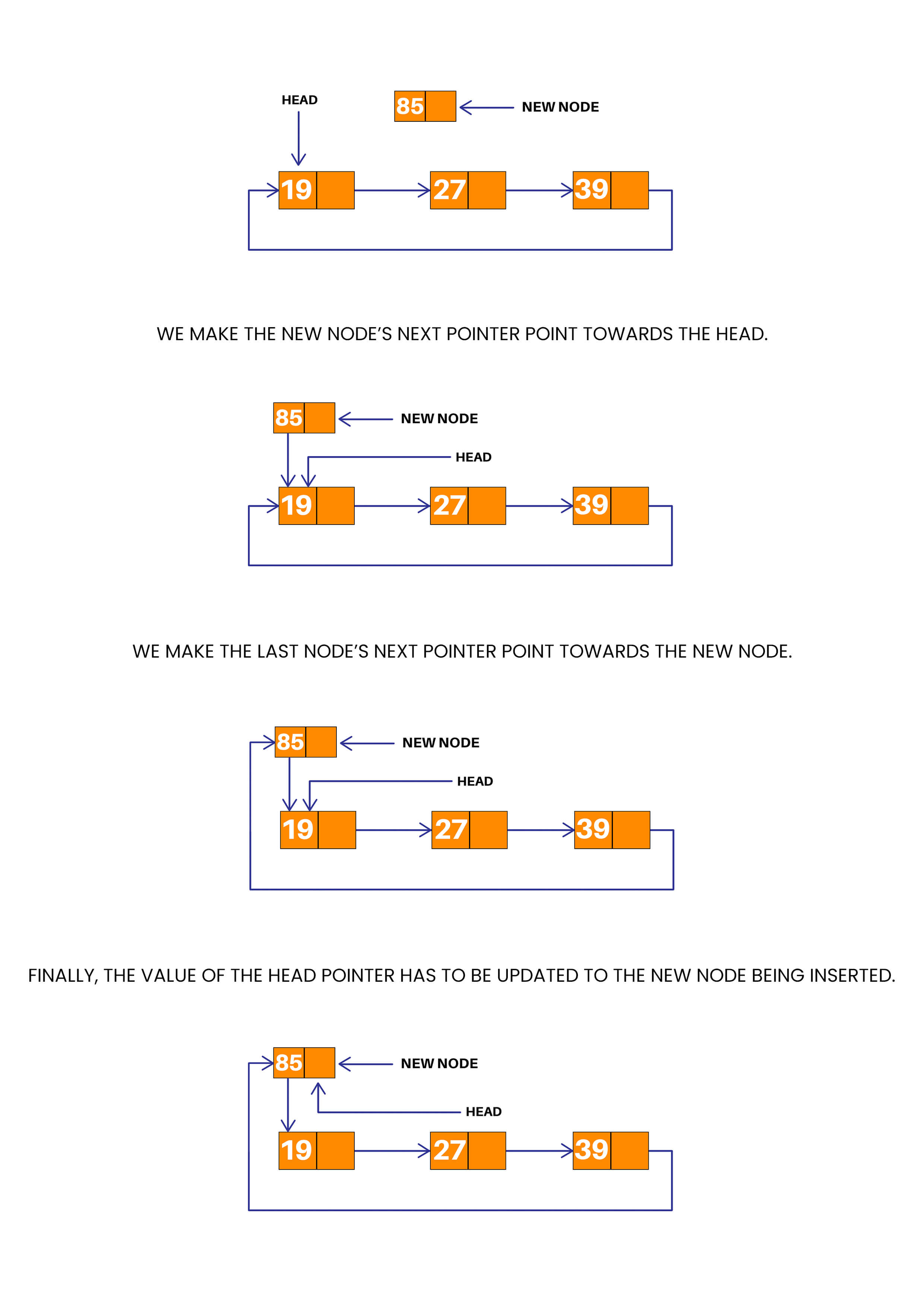Code:

```struct Node *Start(struct Node *head, int data)
{
struct Node *temp = (struct Node *) malloc(sizeof(struct Node));
{
temp -> data = data;
}
else
{
temp -> data = data;
temp -> next = head -> next;
}
}
struct Node *Start(struct Node *head, int data)
{
struct Node *temp = (struct Node *) malloc(sizeof(struct Node));
{
temp -> data = data;
}
else
{
temp -> data = data;
temp -> next = head -> next;
}
}
```

### Insertion at the end

Entering data at the end is much easier than entering data at the beginning. Once again, we need to create a new node and assign the data to it. Following that, we need to traverse to the last node of the linked list.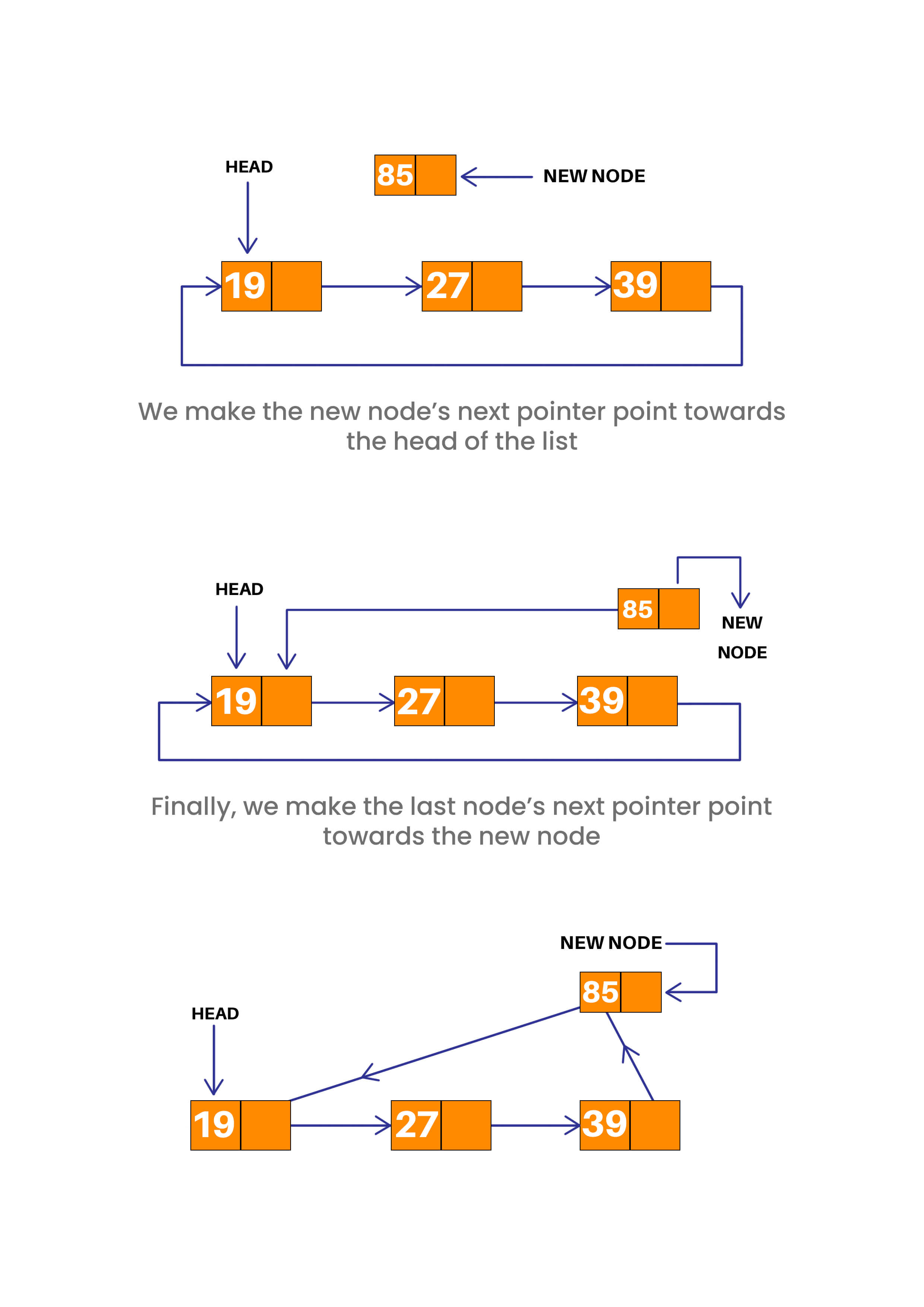Code:

```struct Node *End(struct Node *head, int data)
{
struct Node *temp = (struct Node *) malloc(sizeof(struct Node)), *a = head;
{
temp -> data = data;
}
else
{
do
{
a = a -> next;
} while (a -> next != head);
temp -> data = data;
temp -> next = a -> next;
a -> next = temp;
}
}
```

### Insertion at the middle

The fundamental algorithm for insertion is followed here for generalizing the use cases. Traverse the linked list till before the point where the new node has to be inserted.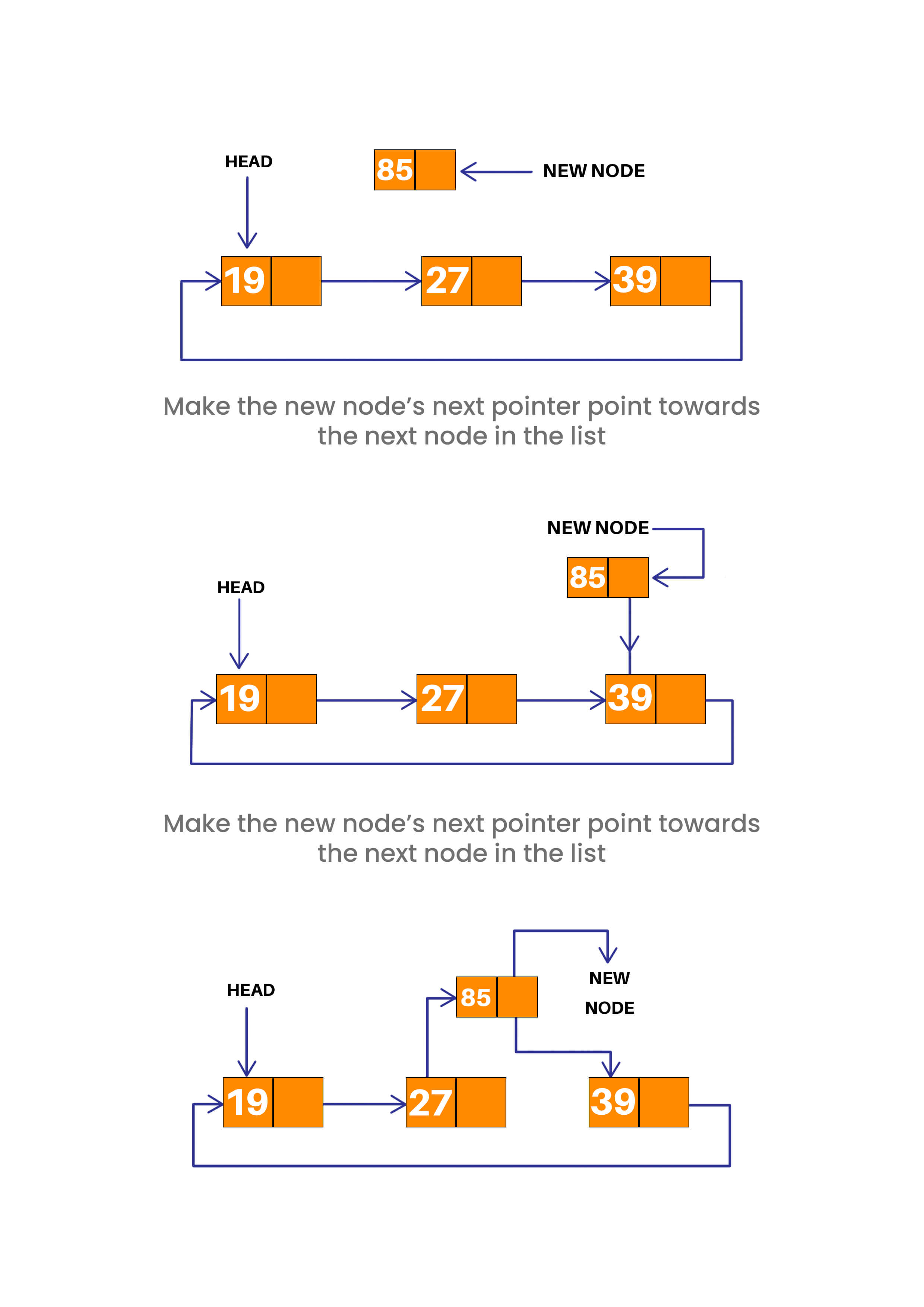Code:

```struct Node *Middle(struct Node *head, int data, int index)
{
{
cout << "List is empty!" << endl;
return NULL;
}

if (index > len || index < 0)
{
cout << "Wrong input, insertion not possible!" << endl;
}
if (index == 0)
{
}
if (index == len)
{
}
struct Node *temp = (struct Node *)malloc(sizeof(struct Node)), *a = head;
do
{
a = a -> next;
} while (a -> next != head);
len = 0;
while (1)
{
if (len == index)
{
temp -> data = data;
temp -> next = a -> next;
a -> next = temp;
}
a = a -> next;
len++;
}
}
```

Similar to insertion, nodes can be deleted either from the beginning, from the end, or from anywhere in between. The only difference here is that one needs to check if the circular linked list is already empty before the deletion or not, which would mean an underflow has occurred, and a suitable error should be displayed.

### Deletion of Element at Start

The process for deletion works almost in a similar manner as for insertion, with only one fundamental difference – deletion requires two pointers, one for pointing towards the node before, and one for pointing towards the node after. In a similar fashion as insertion, traverse till the last node of the linked list with one pointer and store the head of the linked list in another pointer.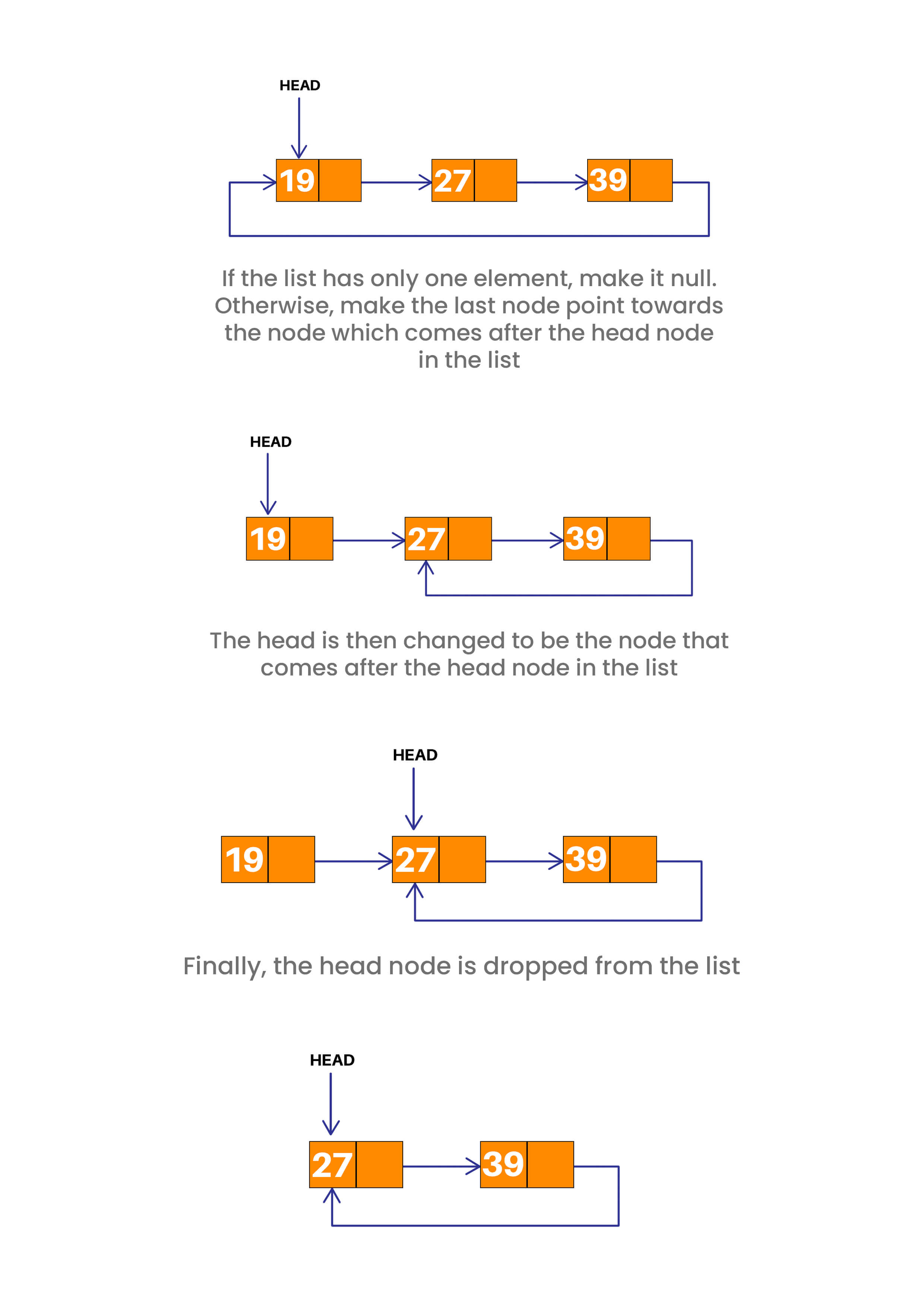Code:

```struct Node *First(struct Node* head)
{

cout << "List is empty" << endl;
return NULL;
}

if (prev->next == prev)
{
}

{
prev = prev->next;
}

prev->next = first->next;

free(first);
}
```

### Deletion of Element at the End

Deletion from the end is considerably easier. All you need to do is traverse till the end of the linked list with one pointer and till the node before the last node with another pointer.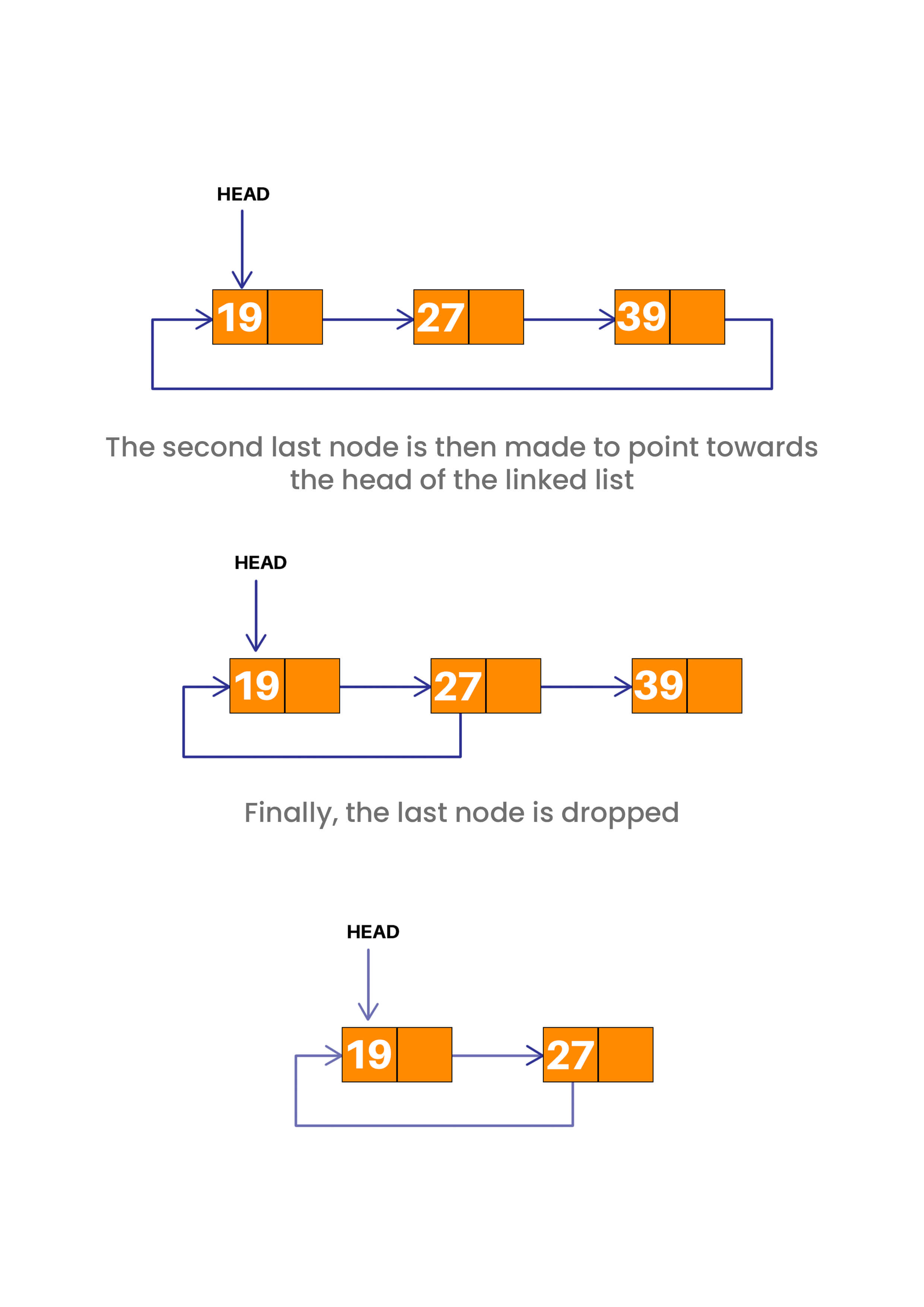Code:

```struct Node *Last(struct Node* head)
{

cout << "List is empty" << endl;
return NULL;
}

if (curr->next == curr)
{
}

{
prev = curr;
curr = curr->next;
}

prev->next = curr->next;
free(curr);
}
```

### Deletion of Element at the Middle

A generalized algorithm for deletion can be designed similarly to insertion. All one needs to do is make one pointer traverse to a node before the required node in the list and one pointer traverse to the required node itself.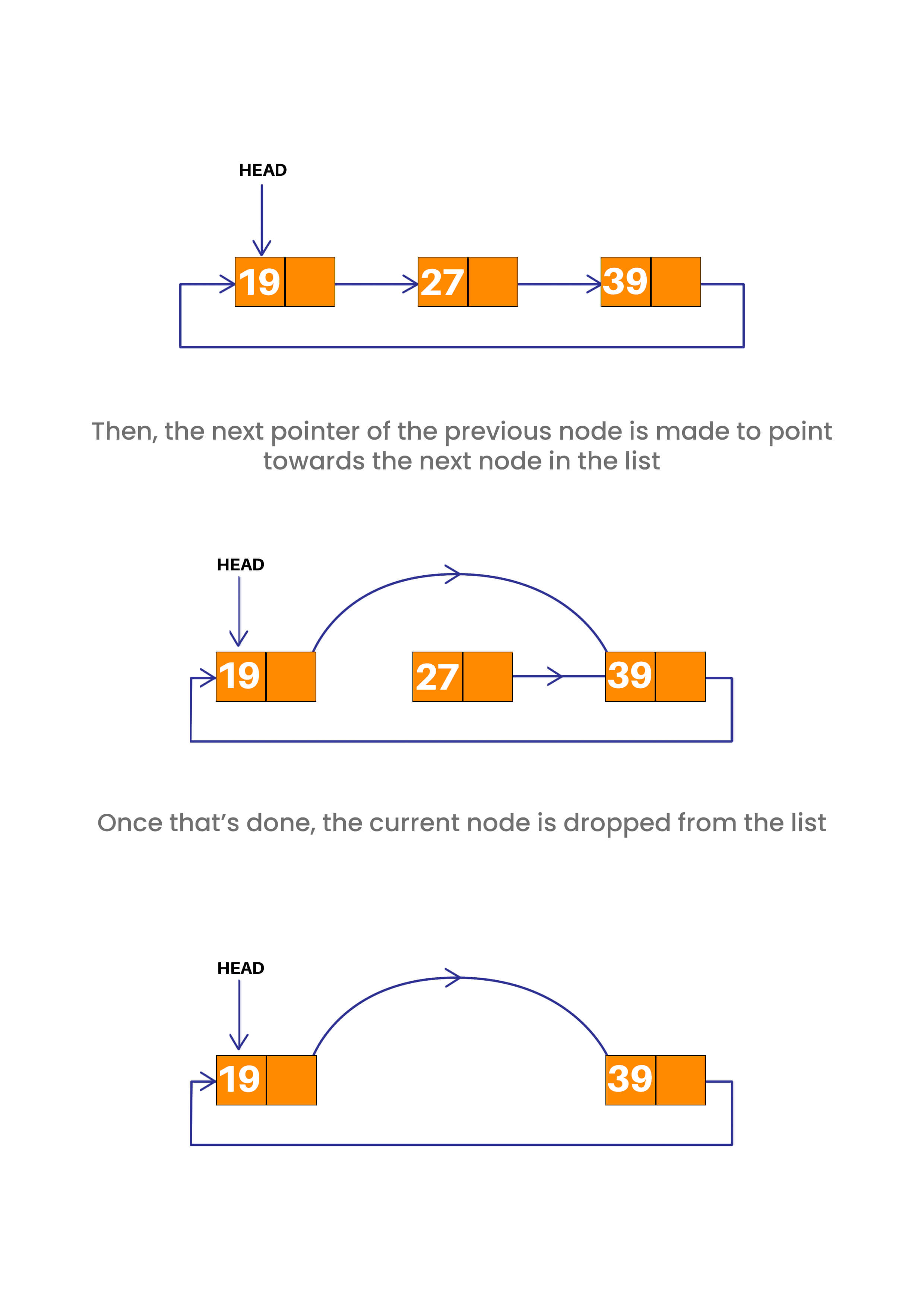Code:

```struct Node *Position(struct Node* head, int index)
{
int count = 1;

cout << "List is empty" << endl;
return NULL;
}

if (index > len || index < 0)
{
cout << "Wrong data given, deletion not possible!" << endl;
}

if (index == 0)
{
}

if (index == len)
{
}

while (len > 0)
{
if (index == count)
{
prev->next = next->next;
free(next);
}
prev = prev->next;
next = prev->next;
len--;
count++;
}

}
```

Perhaps one of the simplest operations in the case of any circular linked list is to print the value of the list. Store the value of the head of the list in a pointer, then traverse through the array till the next pointer of a node points towards the head of the list (that is the main condition for a circular linked list!).

Code:

```void Display(struct Node *head)
{
struct Node *t;
{
cout << "Linked list is empty." << endl;
return;
}

do
{
cout << t -> data << " -> ";
t = t -> next;
cout<<" "<<endl;
}
```

### Circular Linked Lists Using Classes

Here is the code for circular linked lists using class in C++:

```#include <iostream>
using namespace std;

class Node {
public:
int data;
Node* next;
};

private:
Node* tail;
public:
tail = nullptr;
}

Node* newNode = new Node();
newNode->data = val;
tail = newNode;
}
else {
tail->next = newNode;
tail = newNode;
}
}

void displayList() {
cout << "List is empty" << endl;
return;
}
cout << "Nodes of the Circular Linked List: " << endl;
do {
cout << current->data << " ";
current = current->next;
cout << endl;
}
};

int main() {
list.displayList();
return 0;
}
```

Output:

```Nodes of the Circular Linked List:
1 2 3 4
```

We have implemented a circular linked list using two classes. The first class is the Node class. The Node class has no methods and only two properties or class variables. The first one is data to hold the data of the node. The second is a pointer variable to the next Node.

Next, we created another class called the CircularLinkedList. This class is our implementation for the circular linked list codes we have seen so far. In this class, we have two methods and two class variables. The class variables are pointers that store the head and tail of the circular linked list.

The first method is the addNode method. This method takes a value from the user and inserts it at the end of the linked list. This method can be modified with the codes provided above to make the insertion either in the middle or at the beginning.

The last method in the class is the displayList method. This method starts the head and prints all the elements that are present in the Circular Linked List. If the list does not have any element, it prints List is empty.

1. There is no requirement for the NULL pointer in the circular linked list.
2. End operations are well performed in this linked list as the beginning and endpoints are the same.
3. It performs all the operations of a singly linked list properly.
4. Any node in a circular linked list can be considered to be the starting point
5. It saves time when we have to travel from the first node to the last node.

But there are some disadvantages as well. are some of its disadvantages as well. First of all, Each node in a circular linked list requires an extra pointer to maintain the circularity of the list. There are also chances of occurring an infinite loop while dealing with them. But most importantly, reversing a circular linked list is difficult because each node is updated points to the previous node instead of the next node.

But when is a circular linked list actually used in software development? Here are some of the applications:

1. It is easy to implement the scheduling algorithm with the help of a circular linked list.
2. It is used in token ring scheduling algorithms in computer networks.
3. It is easy to implement queue and stack using it.
4. It is used in multiplayer games.
5. It is used to perform the undo functionality in MS Word or Photoshop.

## Conclusion

That concludes our implementation of the circular linked list in C++, how to insert, delete and display nodes. A circular linked list is used in a variety of applications where flexibility is very important. They are used in a variety of practical real-world applications that works with the help of a queue. Happy Learning :)

### FavTutor - 24x7 Live Coding Help from Expert Tutors!